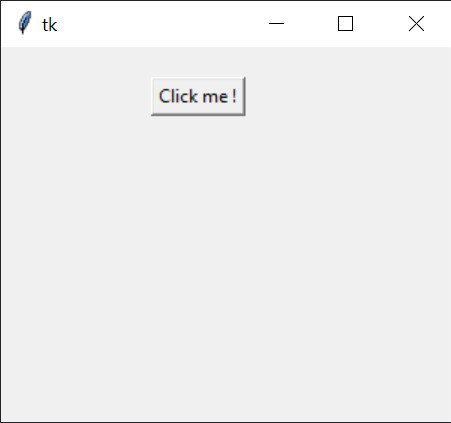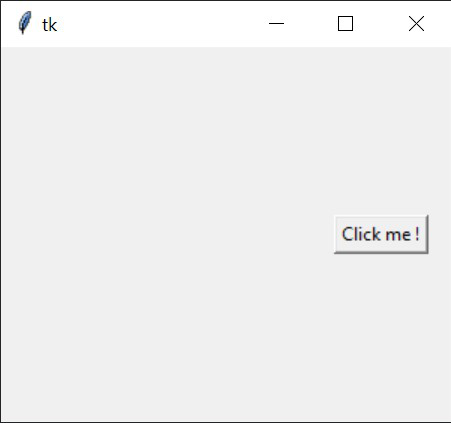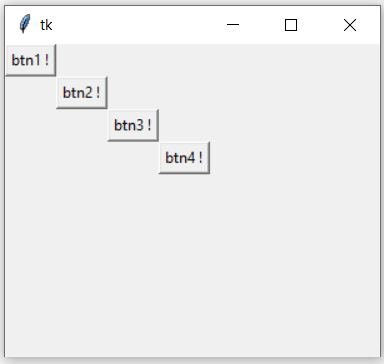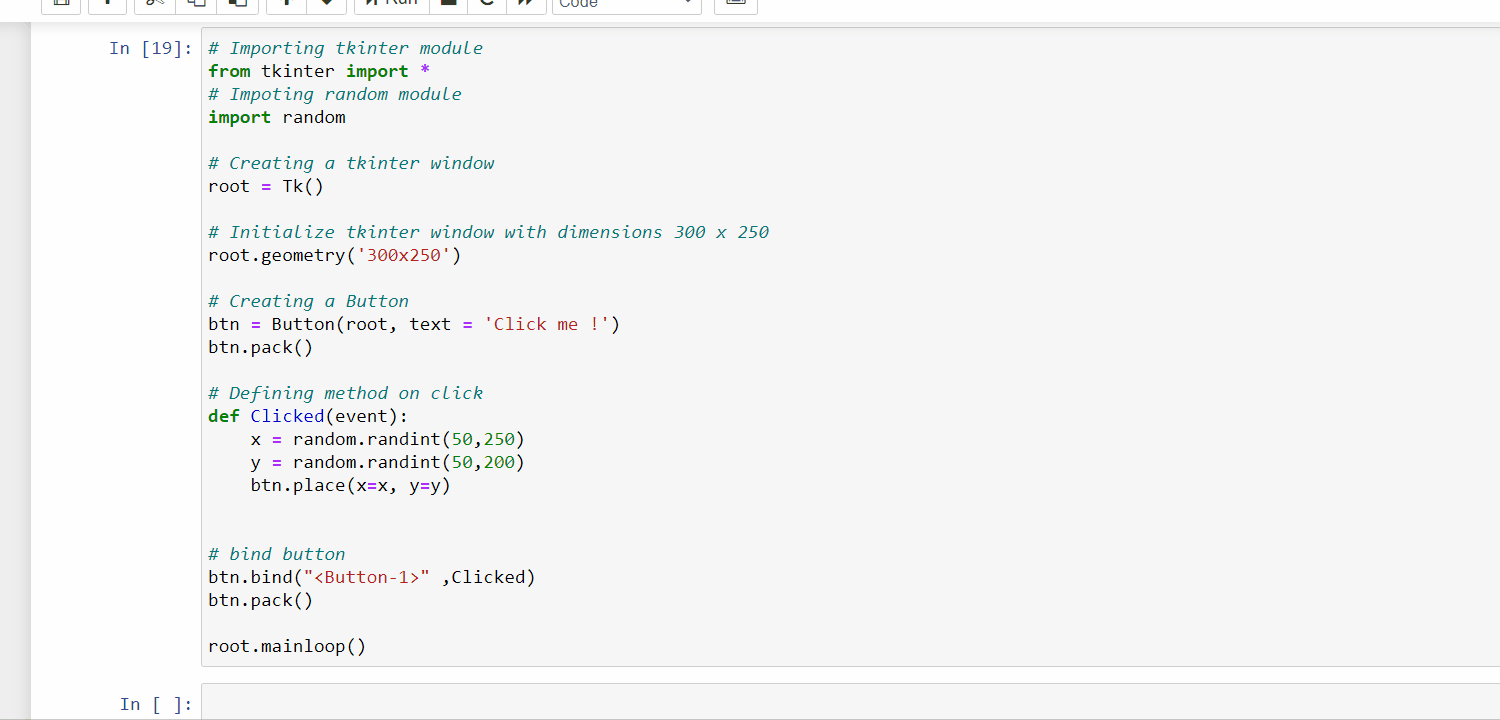GeeksforGeeks App
Open AppBrowser
Continue

# How to move a Tkinter button?

Prerequisite: Creating a button in tkinter

Tkinter is the most commonly used library for developing GUI (Graphical User Interface) in Python. It is a standard Python interface to the Tk GUI toolkit shipped with Python. As Tk and Tkinter are available on most of the Unix platforms as well as on the Windows system, developing GUI applications with Tkinter becomes the fastest and easiest.

This module does not come built-in with Python. To install this module type the below pip command in the terminal.

`pip install tkintertable`

Approach:

• Import the tkinter module # Tkinter in Python 2.x. (Note Capital T).
• Create main window (root = Tk()).
• Add a button to the window.
• Place the button.

The button in the Tkinter module can be placed or move to any position in two ways:

• By using the place method.
• And by using the pack method.

Method 1: Using the place method

This method is used to place a button at an absolute defined position.

Syntax : button1.place(x=some_value, y=some_value)

Parameters :

• x : It defines the x-coordinate of the button’s position.
• y : It defines the y-coordinate of the button’s position.

Below is the implementation of the approach shown above:

## Python3

 `# Importing tkinter module``from` `tkinter ``import` `*`        `# Creating a tkinter window``root ``=` `Tk()` `# Initialize tkinter window with dimensions 300 x 250            ``root.geometry(``'300x250'``)    ` `# Creating a Button``btn ``=` `Button(root, text ``=` `'Click me !'``, command ``=` `root.destroy)` `# Set the position of button to coordinate (100, 20)``btn.place(x``=``100``, y``=``20``)` `root.mainloop()`

Output:Method 2: Using the pack method

This method is used to place a button at a relative position.

Parameters :

• side : It defines the side where the button will be placed.
• padx : It defines the padding on x-axis from the defined side.
• pady : It defines the padding on y-axis from the defines side.

Below is the implementation of the approach shown above:

## Python3

 `# Importing tkinter module``from` `tkinter ``import` `*`        `# Creating a tkinter window``root ``=` `Tk()` `# Initialize tkinter window with dimensions 300 x 250            ``root.geometry(``'300x250'``)    ` `# Creating a Button``btn ``=` `Button(root, text ``=` `'Click me !'``, command ``=` `root.destroy)` `# Set a relative position of button``btn.pack(side``=``RIGHT, padx``=``15``, pady``=``20``)` `root.mainloop()`

Output:Method 3: Using the grid method

This method is used to grid a button at a relative position.

Syntax : button.widget.grid( grid_options )

Parameters :

• column − The column to place widget in; default 0 (leftmost column).
• columnspan − How many columns widgetoccupies; default 1.
• row − The row to put widget in; default the first row that is still empty.
• rowspan − How many rowswidget occupies; default 1.
• sticky − What to try to to if the cell is larger than widget. By default, with sticky=”, widget is centered in its cell. sticky may be the string concatenation of zero or more of N, E, S, W, NE, NW, SE, and SW, compass directions indicating the sides and corners of the cell to which widget sticks.

Below is the implementation of the approach shown above:

## Python3

 `# Importing tkinter module``from` `tkinter ``import` `*`        `# Creating a tkinter window``root ``=` `Tk()` `# Initialize tkinter window``# with dimensions 300 x 250            ``root.geometry(``'300x250'``)    ` `# Creating a Button``btn1 ``=` `Button(root, text ``=` `'btn1 !'``,``              ``command ``=` `root.destroy)``btn1.grid(row ``=` `0``, column ``=` `0``)` `# Creating a Button``btn2 ``=` `Button(root, text ``=` `'btn2 !'``, command ``=` `root.destroy)``btn2.grid(row ``=` `1``, column ``=` `1``)` `# Creating a Button``btn3 ``=` `Button(root, text ``=` `'btn3 !'``, command ``=` `root.destroy)``btn3.grid(row ``=` `2``, column ``=` `2``)` `# Creating a Button``btn3 ``=` `Button(root, text ``=` `'btn4 !'``, command ``=` `root.destroy)``btn3.grid(row ``=` `4``, column ``=` `4``)` `root.mainloop()`

Output:Below is a simple Application to showcase making button movement randomly in a tkinter GUI:

## Python3

 `# Importing tkinter module``from` `tkinter ``import` `*``# Importing random module``import` `random` `# Creating a tkinter window``root ``=` `Tk()` `# Initialize tkinter window with dimensions 300 x 250``root.geometry(``'300x250'``)` `# Creating a Button``btn ``=` `Button(root, text ``=` `'Click me !'``)``btn.pack()` `# Defining method on click``def` `Clicked(event):``    ``x ``=` `random.randint(``50``,``250``)``    ``y ``=` `random.randint(``50``,``200``)``    ``btn.place(x``=``x, y``=``y)``    ` `    ` `# bind button``btn.bind(``""` `,Clicked)``btn.pack()` `root.mainloop()`

Output:My Personal Notes arrow_drop_up